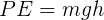physics
Gravitational EnergyGravitational Energy Mass Gravity HeightMass
 g | kg | t
Gravity
 m/s²
Height
 cm | m | km
Gravitational Energy
 J | kCal
Gravity
 m/s²
Height
 cm | m | km
Gravitational Energy
 J | kCal
Mass
 g | kg | t
Height
 cm | m | km
Gravitational Energy
 J | kCal
Gravity
 m/s²
Mass
 g | kg | tgravitational-energy-calculator

en